## Clipping:

When we have to display a large portion of the picture, then not only scaling & translation is necessary, the visible part of picture is also identified. This process is not easy. Certain parts of the image are inside, while others are partially inside. The lines or elements which are partially visible will be omitted. For deciding the visible and invisible portion, a particular process called clipping is used. Clipping determines each element into the visible and invisible portion. Visible portion is selected. An invisible portion is discarded.

### Types of Lines:

Lines are of three types:

1. Visible: A line or lines entirely inside the window is considered visible
2. Invisible: A line entirely outside the window is considered invisible
3. Clipped: A line partially inside the window and partially outside is clipped. For clipping point of intersection of a line with the window is determined.Clipping can be applied through hardware as well as software. In some computers, hardware devices automatically do work of clipping. In a system where hardware clipping is not available software clipping applied.

Following figure show before and after clippingThe window against which object is clipped called a clip window. It can be curved or rectangle in shape.

### Applications of clipping:

1. It will extract part we desire.
2. For identifying the visible and invisible area in the 3D object.
3. For creating objects using solid modeling.
4. For drawing operations.
5. Operations related to the pointing of an object.
6. For deleting, copying, moving part of an object.

Clipping can be applied to world co-ordinates. The contents inside the window will be mapped to device co-ordinates. Another alternative is a complete world co-ordinates picture is assigned to device co-ordinates, and then clipping of viewport boundaries is done.

### Types of Clipping:

1. Point Clipping
2. Line Clipping
3. Area Clipping (Polygon)
4. Curve Clipping
5. Text Clipping
6. Exterior Clipping

### Point Clipping:

Point Clipping is used to determining, whether the point is inside the window or not. For this following conditions are checked.

1. x ≤ xmax
2. x ≥ xmin
3. y ≤ ymax
4. y ≥ yminThe (x, y) is coordinate of the point. If anyone from the above inequalities is false, then the point will fall outside the window and will not be considered to be visible.

### Program1:

To implement Point Clipping:

1. #include<stdio.h>
2. #include<conio.h>
3. #include<graphics.h>
4. inttlx,tly,brx,bry,px,py;
5. void point_clip()
6. {
7. intwxmin,wymin,wxmax,wymax;
8. wxmin=tlx;
9. wxmax=brx;
10. wymin=tly;
11. wymax=bry;
12. if(px>=wxmin&&px<=wxmax)
13. if(py>=wymin&&py<=wymax)
14. putpixel(px,py,RED);
15. getch();
16. closegraph();
17. }
18. void main()
19. {
20. intgd=DETECT,gm,xc,yc,r;
21. clrscr();
22. printf(“Enter the top left coordinate”);
23. scanf(“%d%d”,&tlx,&tly);
24. printf(“Enter the bottom right coordinate”);
25. scanf(“%d%d”,&brx,&bry);
26. printf(“\n Enter the point”);
27. scanf(“%d%d”,&px,&py);
28. initgraph(&gd,&gm,“c:\\tc\\bgi”);
29. setbkcolor(BLUE);
30. setcolor(RED);
31. rectangle(tlx,tly,brx,bry);
32. point_clip();
33. }

Output:### Line Clipping:

It is performed by using the line clipping algorithm. The line clipping algorithms are:

1. Cohen Sutherland Line Clipping Algorithm
2. Midpoint Subdivision Line Clipping Algorithm
3. Liang-Barsky Line Clipping Algorithm

### Cohen Sutherland Line Clipping Algorithm:

In the algorithm, first of all, it is detected whether line lies inside the screen or it is outside the screen. All lines come under any one of the following categories:

1. Visible
2. Not Visible
3. Clipping Case

1. Visible: If a line lies within the window, i.e., both endpoints of the line lies within the window. A line is visible and will be displayed as it is.

2. Not Visible: If a line lies outside the window it will be invisible and rejected. Such lines will not display. If any one of the following inequalities is satisfied, then the line is considered invisible. Let A (x1,y2) and B (x2,y2) are endpoints of line.
3. Clipping Case: If the line is neither visible case nor invisible case. It is considered to be clipped case. First of all, the category of a line is found based on nine regions given below. All nine regions are assigned codes. Each code is of 4 bits. If both endpoints of the line have end bits zero, then the line is considered to be visible.The center area is having the code, 0000, i.e., region 5 is considered a rectangle window.

Following figure show lines of various typesLine AB is the visible case
Line OP is an invisible case
Line PQ is an invisible line
Line IJ are clipping candidates
Line MN are clipping candidate
Line CD are clipping candidate

### Advantage of Cohen Sutherland Line Clipping:

1. It calculates end-points very quickly and rejects and accepts lines quickly.
2. It can clip pictures much large than screen size.

### Algorithm of Cohen Sutherland Line Clipping:

Step1:Calculate positions of both endpoints of the line

Step2:Perform OR operation on both of these end-points

Step3:If the OR operation gives 0000
Then
line is considered to be visible
else
Perform AND operation on both endpoints
If And ≠ 0000
then the line is invisible
else
And=0000
Line is considered the clipped case.

Step4:If a line is clipped case, find an intersection with boundaries of the window
m=(y2-y1 )(x2-x1)

(a) If bit 1 is “1” line intersects with left boundary of rectangle window
y3=y1+m(x-X1)
where X = Xwmin
where Xwminis the minimum value of X co-ordinate of window

(b) If bit 2 is “1” line intersect with right boundary
y3=y1+m(X-X1)
where X = Xwmax
where X more is maximum value of X co-ordinate of the window

(c) If bit 3 is “1” line intersects with bottom boundary
X3=X1+(y-y1)/m
where y = ywmin
ywmin is the minimum value of Y co-ordinate of the window

(d) If bit 4 is “1” line intersects with the top boundary
X3=X1+(y-y1)/m
where y = ywmax
ywmax is the maximum value of Y co-ordinate of the window

### Example of Cohen-Sutherland Line Clipping Algorithm:

Let R be the rectangular window whose lower left-hand corner is at L (-3, 1) and upper right-hand corner is at R (2, 6). Find the region codes for the endpoints in fig:The region code for point (x, y) is set according to the scheme
Bit 1 = sign (y-ymax)=sign (y-6)         Bit 3 = sign (x-xmax)= sign (x-2)
Bit 2 = sign (ymin-y)=sign(1-y)         Bit 4 = sign (xmin-x)=sign(-3-x)

HereSo

A (-4, 2)→ 0001         F (1, 2)→ 0000
B (-1, 7) → 1000         G (1, -2) →0100
C (-1, 5)→ 0000         H (3, 3) → 0100
D (3, 8) → 1010         I (-4, 7) → 1001
E (-2, 3) → 0000         J (-2, 10) → 1000

We place the line segments in their appropriate categories by testing the region codes found in the problem.

Category1 (visible): EF since the region code for both endpoints is 0000.

Category2 (not visible): IJ since (1001) AND (1000) =1000 (which is not 0000).

Category 3 (candidate for clipping): AB since (0001) AND (1000) = 0000, CD since (0000) AND (1010) =0000, and GH. since (0100) AND (0010) =0000.

The candidates for clipping are AB, CD, and GH.

In clipping AB, the code for A is 0001. To push the 1 to 0, we clip against the boundary line xmin=-3. The resulting

intersection point is I1 (-3,3). We clip (do not display) AI1 and I1 B. The code for I1is 1001. The clipping category for I1 B is 3 since (0000) AND (1000) is (0000). Now B is outside the window (i.e., its code is 1000), so we push the 1

to a 0 by clipping against the line ymax=6. The resulting intersection is l2 (-1,6). Thus I2 B is clipped. The code for I2 is 0000. The remaining segment I1 I2 is displayed since both endpoints lie in the window (i.e., their codes are 0000).

For clipping CD, we start with D since it is outside the window. Its code is 1010. We push the first 1 to a 0 by clipping against the line ymax=6. The resulting intersection I3 is (,6),and its code is 0000. Thus I3 D is clipped and the remaining segment CI3 has both endpoints coded 0000 and so it is displayed.

For clipping GH, we can start with either G or H since both are outside the window. The code for G is 0100, and we push the 1 to a 0 by clipping against the line ymin=1.The resulting intersection point is I4 (2,1) and its code is 0010. We clip GI4 and work on I4 H. Segment I4 H is not displaying since (0010) AND (0010) =0010.

### Program to perform Line Clipping using Cohen Sutherland Algorithm:

1. #include <iostream.h>
#include <conio.h>
#include <graphics.h>
#include <dos.h>
class data
{
int gd, gmode, x, y, xmin,ymin,ymax,xmax;
int a1,a2;
float x1, y1,x2,y2,x3,y3;
int xs, ys, xe, ye;
float maxx,maxy;
public:
void getdata ();
void find ();
void clip ();
void display (float, float,float,float);
void checkonof (int);
void showbit (int);
};
void data :: getdata ()
{
cout<<"Enter the minimum and maximum coordinate of window (x, y) ";
cin >>xmin>>ymin>>xmax>>ymax;
cout<<"Enter the end points of the line to be clipped";
cin >>xs>>ys>>xe>>ye;
display (xs, ys, xe,ye);
}
void data :: display (float, xs, float, ys,float xe, float ye)
{
int gd=DETECT;
initgraph (&gd,&gmode, "");
maxx=getmaxx();
maxy=getmaxy();
line (maxx/2,0,maxx/2,maxy);
line (0, maxy/2,maxx,maxy/2);
rectangle (maxx/2+xmin,maxy/2-ymax,maxx/2+xmax,maxy/2-ymin);
line (maxx/2+xs,maxy/2-ys,maxx/2+xe,maxy/2-ye);
getch();
}
void data :: find ()
{
a1=0;
a2=0;
if ((ys-ymax)>0)
a1+=8;
if ((ymin-ys)>0)
a1+=4;
if ((xs-xmax)>0)
a1+=2;
if ((xmin-xs)>0)
a1+=1;
if ((ye-ymax)>0)
a2+=8;
if ((ymin-ye)>0)
a2+=4;
if ((xe-xmax)>0)
a2+=2;
if ((xmin-xe)>0)
a2+=1;
cout<<"\nThe area code of Ist point is ";
showbit (a1);
getch ();
cout <<"\nThe area code of 2nd point is ";
showbit (a2);
getch ();
}
void data :: showbit (int n)
{
int i,k, and;
for (i=3;i>=0;i--)
{
and =1<<i;
k = n?
k ==0?cout<<"0": cout<<"1\"";
}
}
void data ::clip()
{
int j=a1&a2;
if (j==0)
{
cout<<"\nLine is perfect candidate for clipping";
if (a1==0)
{
else
{
checkonof(a1);
x2=x1;y2=y1;
}
if (a2=0)
{
x3=xe; y3=ye;
}
else
{
checkonof (a2);
x3=x1; y3=y1;
}
xs=x2; ys=y2;xe=x3;ye=y3;
cout << endl;
display (xs,ys,xe,ye);
cout<<"Line after clipping";
getch ()
}
else if ((a1==0) && (a2=0))
{
cout <<"\n Line is in the visible region";
getch ();
}
}
void data :: checkonof (int i)
{
int j, k,l,m;
1=i&1;
x1=0;y1=0;
if (1==1)
{
x1=xmin;
y1=ys+ ((x1-xs)/ (xe-xs))*(ye-ys);
}
j=i&8;
if (j>0)
{
y1=ymax;
x1=xs+(y1-ys)/(ye-ys))*(xe-xs);
}
k=i & 4;
if (k==1)
{
y1=ymin;
x1=xs+((y1-ys)/(ye-ys))*(xe-xs);
}
m= i&2;
if (m==1)
{
x1=xmax;
y1=ys+ ((x1-xs)/ (xe-xs))*(ye-ys);
}
main ()
{
data s;
clrscr();
s.getdata();
s.find();
getch();
closegraph ();
return ();
}

Output:### Mid Point Subdivision Line Clipping Algorithm:

It is used for clipping line. The line is divided in two parts. Mid points of line is obtained by dividing it in two short segments. Again division is done, by finding midpoint. This process is continued until line of visible and invisible category is obtained. Let (xi,yi) are midpointx5lie on point of intersection of boundary of window.

### Advantage of midpoint subdivision Line Clipping:

It is suitable for machines in which multiplication and division operation is not possible. Because it can be performed by introducing clipping divides in hardware.

### Algorithm of midpoint subdivision Line Clipping:

Step1: Calculate the position of both endpoints of the line

Step2: Perform OR operation on both of these endpoints

Step3: If the OR operation gives 0000
then
Line is guaranteed to be visible
else
Perform AND operation on both endpoints.
If AND ≠ 0000
then the line is invisible
else
AND=6000
then the line is clipped case.

Step4: For the line to be clipped. Find midpoint
Xm=(x1+x2)/2
Ym=(y1+y2)/2
Xmis midpoint of X coordinate.
Ymis midpoint of Y coordinate.

Step5: Check each midpoint, whether it nearest to the boundary of a window or not.

Step6: If the line is totally visible or totally rejected not found then repeat step 1 to 5.

Step7: Stop algorithm.

Example: Window size is (-3, 1) to (2, 6). A line AB is given having co-ordinates of A (-4, 2) and B (-1, 7). Does this line visible. Find the visible portion of the line using midpoint subdivision?

Solution:

Step1: Fix point A (-4, 2)Step2: Find b”=mid of b’and bSo (-1, 5) is better than (2, 4)
Find b”&bb”(-1, 5) b (-1, 7)So B””to B length of line will be clipped from upper side

Now considered left-hand side portion.

A and B””are now endpoints

Find mid of A and B””

A (-4, 2) B “”(-1, 6)### Liang-Barsky Line Clipping Algorithm:

Liang and Barsky have established an algorithm that uses floating-point arithmetic but finds the appropriate endpoints with at most four computations. This algorithm uses the parametric equations for a line and solves four inequalities to find the range of the parameter for which the line is in the viewport.Let P(x1, y1), Q(x2, y2) is the line which we want to study. The parametric equation of the line segment from gives x-values and y-values for every point in terms of a parameter that ranges from 0 to 1. The equations are

x=x1+(x2-x1 )*t=x1+dx*t and y=y1+(y2-y1 )*t=y1+dy*t

We can see that when t = 0, the point computed is P(x1, y1); and when t = 1, the point computed is Q(x2, y2).

### Algorithm of Liang-Barsky Line Clipping:

1. Set tmin=0 and tmax=1

2. Calculate the values tL,tR,tT and tB(tvalues).
If t<tmin or t<tmax? ignore it and go to the next edge
Otherwise classify the tvalue as entering or exiting value (using inner product to classify)
If t is entering value set tmin=t if t is exiting value set tmax=t.</t</t

3. If tmin< tmax? then draw a line from (x1 + dx*tmin, y1 + dy*tmin) to (x1 + dx*tmax?, y1 + dy*tmax? )

4. If the line crosses over the window, you will see (x1 + dx*tmin, y1 + dy*tmin) and (x1 + dx*tmax? , y1 + dy*tmax?) are intersection between line and edge.

### Text Clipping:

Several methods are available for clipping of text. Clipping method is dependent on the method of generation used for characters. A simple method is completely considered, or nothing considers method. This method is also called as all or none. If all characters of the string are inside window, then we will keep the string, if a string character is outside then whole string will be discarded in fig (a). Another method is discarded those characters not completely inside the window. If a character overlap boundary of window. Those will be discarded in fig (b).In fig (c) individual character is treated. Character lies on boundary is discarded as which it is outside the window.

### Curve Clipping:

Curve Clipping involves complex procedures as compared to line clipping. Curve clipping requires more processing than for object with linear boundaries. Consider window which is rectangular in shape. The circle is to consider against rectangle window. If circle is completely inside boundary of the window, it is considered visible. So save the circle. If a circle is in outside window, discard it. If circle cut the boundary then consider it to be clipping case.

### Exterior Clipping:

It is opposite to previous clipping. Here picture which is outside the window is considered. The picture inside the rectangle window is discarded. So part of the picture outside the window is saved.

Uses of Exterior Clipping:

1. It is used for displaying properly the pictures which overlap each other.
2. It is used in the concept of overlapping windows.
3. It is used for designing various patterns of pictures.
4. It is used for advertising purposes.
5. It is suitable for publishing.
6. For designing and displaying of the number of maps and charts, it is also used.

### Polygon Clipping:

Polygon clipping is applied to the polygons. The term polygon is used to define objects having outline of solid. These objects should maintain property and shape of polygon after clipping.

### Polygon:

Polygon is a representation of the surface. It is primitive which is closed in nature. It is formed using a collection of lines. It is also called as many-sided figure. The lines combined to form polygon are called sides or edges. The lines are obtained by combining two vertices.

### Example of Polygon:

1. Triangle
2. Rectangle
3. Hexagon
4. Pentagon

Following figures shows some polygons.### Types of Polygons

1. Concave
2. Convex

A polygon is called convex of line joining any two interior points of the polygon lies inside the polygon. A non-convex polygon is said to be concave. A concave polygon has one interior angle greater than 180°. So that it can be clipped into similar polygons.A polygon can be positive or negative oriented. If we visit vertices and vertices visit produces counterclockwise circuit, then orientation is said to be positive.### Sutherland-Hodgeman Polygon Clipping:

It is performed by processing the boundary of polygon against each window corner or edge. First of all entire polygon is clipped against one edge, then resulting polygon is considered, then the polygon is considered against the second edge, so on for all four edges.

Four possible situations while processing

1. If the first vertex is an outside the window, the second vertex is inside the window. Then second vertex is added to the output list. The point of intersection of window boundary and polygon side (edge) is also added to the output line.
2. If both vertexes are inside window boundary. Then only second vertex is added to the output list.
3. If the first vertex is inside the window and second is an outside window. The edge which intersects with window is added to output list.
4. If both vertices are the outside window, then nothing is added to output list.

Following figures shows original polygon and clipping of polygon against four windows.### Disadvantage of Cohen Hodgmen Algorithm:

This method requires a considerable amount of memory. The first of all polygons are stored in original form. Then clipping against left edge done and output is stored. Then clipping against right edge done, then top edge. Finally, the bottom edge is clipped. Results of all these operations are stored in memory. So wastage of memory for storing intermediate polygons.### Weiler-Atherton Polygon Clipping:

Let the clipping window be initially called clip polygon and the polygon to be clipped the subject polygon. We start with an arbitrary vertex of the subject polygon and trace around its border in the clockwise direction until an intersection with the clip polygon is encountered:

1. If the edge enters the clip polygon, record the intersection point and continue to trace the subject polygon.2. If the edge leaves the clip polygon, record the intersection point and make a right turn to follow the clip polygon in the same manner (i.e., treat the clip polygon as subject polygon and the subject polygon as clip polygon and proceed as before).

Whenever our path of traversal forms a sub-polygon we output the sub-polygon as part of the overall result. We then continue to trace the rest of the original subject polygon from a recorded intersection point that marks the beginning of a not-yet traced edge or portion of an edge. The algorithm terminates when the entire border of the original subject polygon has been traced exactly once.For example, the number in fig (a) indicates the order in which the edges and portion of edges are traced. We begin at the starting vertex and continue along the same edge (from 1 to 2) of the subject polygon as it enters the clip polygon. As we move along the edge that is leaving the clip polygon, we make a right turn (from 4 to 5) onto the clip polygon, which is now considered the subject polygon. Following the same logic leads to the next right turn (from 5 to 6) onto the current clip polygon, this is the original subject polygon. With the next step done (from 7 to 8) in the same way, we have a sub-polygon for output in fig (b). We then resume our traversal of the original subject polygon from the recorded intersection point where we first changed our course. Going from 9 to 10 to 11 produces no output. After skipping the already traversed 6 and 7, we continue with 12 and 13 and come to an end. The fig (b) is the final result.## Scan Converting a Ellipse:

The ellipse is also a symmetric figure like a circle but is four-way symmetry rather than eight-way.### Program to Implement Ellipse Drawing Algorithm:

1. #include<stdio.h>
#include<conio.h>
#include<graphics.h>
#include<math.h>
void display();
float x,y;
int xc,yc;
int main()
{
int gd=DETECT,gm,a,b;
float p1,p2;
//clrscr();
initgraph(&gd,&gm,"c:\\turboc3\\bgi");
printf(" Ellipse Generating Algorithm \n\n");
printf("Enter the value of Xc\t");
scanf("%d",&xc);
printf("Enter the value of Yc\t");
scanf("%d",&yc);
printf("Enter X axis length\t");
scanf("%d",&a);
printf("Enter Y axis length\t");
scanf("%d",&b);
x=0;y=b;
display();
p1=(b*b)-(a*a*b)+(a*a)/4;
while((2.0*b*b*x)<=(2.0*a*a*y))
{
x++;
if(p1<=0)
p1=p1+(2.0*b*b*x)+(b*b);
else
{
y--;
p1=p1+(2.0*b*b*x)+(b*b)-(2.0*a*a*y);
}
display();
x=-x;
display();
x=-x;
delay(50);
}
x=a;
y=0;
display();
p2=(a*a)+2.0*(b*b*a)+(b*b)/4;
while((2.0*b*b*x)>(2.0*a*a*y))
{
y++;
if(p2>0)
p2=p2+(a*a)-(2.0*a*a*y);
else
{
x--;
p2=p2+(2.0*b*b*x)-(2.0*a*a*y)+(a*a);
}
display();
y=-y;
display();
y=-y;
delay(50);
}
getch();
closegraph();
}
void display()
{
putpixel(xc+x,yc+y,7);
putpixel(xc-x,yc+y,7);
putpixel(xc+x,yc-y,7);
putpixel(xc+x,yc-y,7);
}

Output: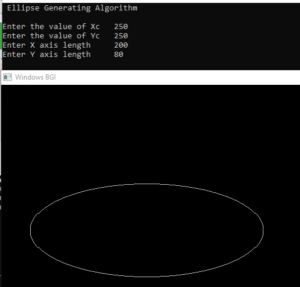There two methods of defining an Ellipse:

1. Polynomial Method of defining an Ellipse
2. Trigonometric method of defining an Ellipse

### Polynomial Method:

The ellipse has a major and minor axis. If a1 and b1are major and minor axis respectively. The centre of ellipse is (i, j). The value of x will be incremented from i to a1and value of y will be calculated using the following formula### Drawback of Polynomial Method:

1. It requires squaring of values. So floating point calculation is required.
2. Routines developed for such calculations are very complex and slow.### Algorithm:

1. Set the initial variables: a = length of major axis; b = length of minor axis; (h, k) = coordinates of ellipse center; x = 0; i = step; xend = a.

2. Test to determine whether the entire ellipse has been scan-converted. If x>xend, stop.

3. Compute the value of the y coordinate:4. Plot the four points, found by symmetry, at the current (x, y) coordinates:

Plot (x + h, y + k)           Plot (-x + h, -y + k)           Plot (-y – h, x + k)           Plot (y + h, -x + k)

5. Increment x; x = x + i.

6. Go to step 2.

### Program to draw an Ellipse using Polynomial Method:

#include <graphics.h>
#include <stdlib.h>
#include <math.h>
#include <stdio.h>
#include <conio.h>
#include <iostream>
using namespace std;
class bresen
{
float x, y, a, b, r, t, te, xend, h, k, step;
public:
void get ();
void cal ();
};
int main ()
{
bresen b;
b.get ();
b.cal ();
getch ();
}
void bresen :: get ()
{
cout<<"\n ENTER CENTER OF ELLIPSE";
cout<<"\n enter (h, k) ";
cin>>h>>k;
cout<<"\n ENTER LENGTH OF MAJOR AND MINOR AXIS";
cin>>a>>b;
cout<<"\n ENTER Step Size";
cin>> step;
}
void bresen ::cal ()
{
/* request auto detection */
int gdriver = DETECT,gmode, errorcode;
int midx, midy, i;
/* initialize graphics and local variables */
initgraph (&gdriver, &gmode, " ");
/* read result of initialization */
errorcode = graphresult ();
if (errorcode != grOk) /* an error occurred */
{
printf("Graphics error: %s\n", grapherrormsg(errorcode));
printf("Press any key to halt:");
getch();
exit(1); /* terminate with an error code */
}
x = 0;
xend=a;
while (x<xend)
{
t= (1-((x * x)/ (a * a)));
if (t<0)
te=-t;
else
te=t;
y=b * sqrt (te);
putpixel (h+x, k+y, RED);
putpixel (h-x, k+y, RED);
putpixel (h+x, y-y, RED);
putpixel (h-x, k-y, RED);
x+=step;
}
getch();
}


Output:### Trignometric Method:

The following equation defines an ellipse trigonometrically as shown in fig:

x = a * cos (θ) +h and
y = b * sin (θ)+k
where (x, y) = the current coordinates
a = length of major axis
b = length of minor axis
θ= current angle
(h, k) = ellipse center

In this method, the value of θ is varied from 0 toradians. The remaining points are found by symmetry.### Drawback:

1. This is an inefficient method.
2. It is not an interactive method for generating ellipse.
3. The table is required to see the trigonometric value.
4. Memory is required to store the value of θ.

### Algorithm:

Step1: Start Algorithm

Step2: Declare variable x1,y1,aa1,bb1,aa2,bb2,fx,fy,p1,a1,b1

Step3: Initialize x1=0 and y1=b/* values of starting point of circle */

Step4: Calculate aa1=a1*a1
Calculate bb1=b1* b1
Calculate aa2=aa1*2
Calculate bb2=bb1*2

Step5: Initialize fx = 0

Step6: Initialize fy = aa_2* b1

Step7: Calculate the value of p1and round if it is integer
p1=bb1-aa1* b1+0.25* aa1/

Step8:

While (fx < fy)
{
Set pixel (x1,y1)
Increment x i.e., x = x + 1
Calculate fx = fx + bb2
If (p1 < 0)
Calculate p1 = p1 + fx + bb1/
else
{
Decrement y i.e., y = y-1
Calculate fy = fy - 992;
p1=p1 + fx + bb1-fy
}
}

Step9: Setpixel (x1,y1)

Step10: Calculate p1=bb1 (x+.5)(x+.5)+aa(y-1)(y-1)-aa1*bb1

Step 11:

While (y1>0)
{
Decrement y i.e., y = y-1
fy=fx-aa2/
if (p1>=0)
p1=p1 - fx +  aa1/
else
{
Increment x i.e., x = x + 1
fx= fx+bb_2
p1=p1+fx-fy-aa1
}
}
Set pixel (x1,y1)

Step12: Stop Algorithm

### Program to draw a circle using Trigonometric method:

#include <graphics.h>
#include <stdlib.h>
#include <math.h>
#include <stdio.h>
#include <conio.h>
#include <iostream>
# define pi 3.14
using namespace std;
class bresen
{
float a, b, h, k, thetaend,step,x,y;
int i;
public:
void get ();
void cal ();
};
int main ()
{
bresen b;
b.get ();
b.cal ();
getch ();
}
void bresen :: get ()
{
cout<<"\n ENTER CENTER OF ELLIPSE";
cin>>h>>k;
cout<<"\n ENTER LENGTH OF MAJOR AND MINOR AXIS";
cin>>a>>b;
cout<<"\n ENTER STEP SIZE";
cin>> step;
}
void bresen ::cal ()
{
/* request auto detection */
int gdriver = DETECT,gmode, errorcode;
int midx, midy, i;
/* initialize graphics and local variables */
initgraph (&gdriver, &gmode, " ");
/* read result of initialization */
errorcode = graphresult ();
if (errorcode != grOk) /* an error occurred */
{
printf("Graphics error: %s\n", grapherrormsg(errorcode));
printf("Press any key to halt:");
getch();
exit(1); /* terminate with an error code */
}
int theta = 0;
thetaend=(pi*90)/180;
while (theta<thetaend)
{
x = a * cos (theta);
y = b * sin (theta);
putpixel (x+h, y+k, RED);
putpixel (-x+h, y+k, RED);
putpixel (-x+h, -y+k, RED);
putpixel (x+h, -y+k, RED);
theta+=step;
}
getch();
}


Output:### Ellipse Axis Rotation:

Since the ellipse shows four-way symmetry, it can easily be rotated. The new equation is found by trading a and b, the values which describe the major and minor axes. When the polynomial method is used, the equations used to describe the ellipse becomewhere (h, k) = ellipse center
a = length of the major axis
b = length of the minor axis
In the trigonometric method, the equations are
x = b cos (θ)+h       and       y=a sin(θ)+k

Where (x, y) = current coordinates
a = length of the major axis
b = length of the minor axis
θ = current angle
(h, k) = ellipse center

Assume that you would like to rotate the ellipse through an angle other than 90 degrees. The rotation of the ellipse may be accomplished by rotating the x &y axis α degrees.

x = a cos (0) – b sin (0+ ∞) + h y= b (sin 0) + a cos (0+∞) + k### Midpoint Ellipse Algorithm:

This is an incremental method for scan converting an ellipse that is centered at the origin in standard position i.e., with the major and minor axis parallel to coordinate system axis. It is very similar to the midpoint circle algorithm. Because of the four-way symmetry property we need to consider the entire elliptical curve in the first quadrant.

Let’s first rewrite the ellipse equation and define the function f that can be used to decide if the midpoint between two candidate pixels is inside or outside the ellipse:Now divide the elliptical curve from (0, b) to (a, 0) into two parts at point Q where the slope of the curve is -1.

Slope of the curve is defined by the f(x, y) = 0 iswhere fx & fy are partial derivatives of f(x, y) with respect to x & y.

We have fx = 2b2 x, fy=2a2 y &Hence we can monitor the slope value during the scan conversion process to detect Q. Our starting point is (0, b)

Suppose that the coordinates of the last scan converted pixel upon entering step i are (xi,yi). We are to select either T (xi+1),yi) or S (xi+1,yi-1) to be the next pixel. The midpoint of T & S is used to define the following decision parameter.

pi = f(xi+1),yi)
pi=b2 (xi+1)2+a2 (yi)2-a2 b2

If pi<0, the midpoint is inside the curve and we choose pixel T.

If pi>0, the midpoint is outside or on the curve and we choose pixel S.

Decision parameter for the next step is:

pi+1=f(xi+1+1,yi+1)
= b2 (xi+1+1)2+a2 (yi+1)2-a2 b2

Since xi+1=xi+1,we have
pi+1-pi=b2[((xi+1+1)2+a2 (yi+1)2-(yi –)2]
pi+1= pi+2b2 xi+1+b2+a2 [(yi+1)2-(yi –)2]

If T is chosen pixel (pi<0), we have yi+1=yi.

If S is chosen pixel (pi>0) we have yi+1=yi-1. Thus we can express

pi+1in terms of pi and (xi+1,yi+1):           pi+1= pi+2b2 xi+1+b2          if pi<0           = pi+2b2 xi+1+b2-2a2 yi+1 if pi>0

The initial value for the recursive expression can be obtained by the evaluating the original definition of pi with (0, b):

p1 = (b2+a2 (b-)2-a2 b2
= b2-a2 b+a2/4

Suppose the pixel (xj yj) has just been scan converted upon entering step j. The next pixel is either U (xj ,yj-1) or V (xj+1,yj-1). The midpoint of the horizontal line connecting U & V is used to define the decision parameter:

qj=f(xj+,yj-1)
qj=b2 (xj+)2+a2 (yj -1)2-a2 b2

If qj<0, the midpoint is inside the curve and we choose pixel V.

If qj≥0, the midpoint is outside the curve and we choose pixel U.Decision parameter for the next step is:

qj+1=f(xj+1+,yj+1-1)
= b2 (xj+1+)2+ a2 (yj+1-1)2– a2 b2

Since yj+1=yj-1,we have
qj+1-qj=b2 [(xj+1+)2-(xj +)2 ]+a2 (yj+1-1)2-( yj+1)2 ]
qj+1=qj+b2 [(xj+1+)2-(xj +)2]-2a2 yj+1+a2

If V is chosen pixel (qj<0), we have xj+1=xj.

If U is chosen pixel (pi>0) we have xj+1=xj. Thus we can express

qj+1in terms of qj and (xj+1,yj+1 ):
qj+1=qj+2b2 xj+1-2a2 yj+1+a2          if qj < 0
=qj-2a2 yj+1+a2          if qj>0

The initial value for the recursive expression is computed using the original definition of qj. And the coordinates of (xk yk) of the last pixel choosen for the part 1 of the curve:

q1 = f(xk+,yk-1)=b2 (xk+)2-a2 (yk-1)2– a2 b2

### Algorithm:

int x=0, y=b; [starting point]
int fx=0, fy=2a2 b [initial partial derivatives]
int p = b2-a2 b+a2/4
while (fx2;
if (p<0)
p = p + fx +b2;
else
{
y--;
fy=fy-2a2
p = p + fx +b2-fy;
}
}
Setpixel (x, y);
p=b2(x+0.5)2+ a2 (y-1)2- a2 b2
while (y>0)
{
y--;
fy=fy-2a2;
if (p>=0)
p=p-fy+a2
else
{
x++;
fx=fx+2b2
p=p+fx-fy+a2;
}
Setpixel (x,y);
}

### Program to draw an ellipse using Midpoint Ellipse Algorithm:

#include <graphics.h>
#include <stdlib.h>
#include <math.h>
#include <stdio.h>
#include <conio.h>
#include <iostream.h>

class bresen
{
float x,y,a, b,r,p,h,k,p1,p2;
public:
void get ();
void cal ();
};
void main ()
{
bresen b;
b.get ();
b.cal ();
getch ();
}
void bresen :: get ()
{
cout<<"\n ENTER CENTER OF ELLIPSE";
cout<<"\n ENTER (h, k) ";
cin>>h>>k;
cout<<"\n ENTER LENGTH OF MAJOR AND MINOR AXIS";
cin>>a>>b;
}
void bresen ::cal ()
{
/* request auto detection */
int gdriver = DETECT,gmode, errorcode;
int midx, midy, i;
/* initialize graphics and local variables */
initgraph (&gdriver, &gmode, " ");
/* read result of initialization */
errorcode = graphresult ();
if (errorcode ! = grOK)    /*an error occurred */
{
printf("Graphics error: %s \n", grapherrormsg (errorcode);
printf ("Press any key to halt:");
getch ();
exit (1); /* terminate with an error code */
}
x=0;
y=b;
// REGION 1
p1 =(b * b)-(a * a * b) + (a * a)/4);
{
putpixel (x+h, y+k, RED);
putpixel (-x+h, -y+k, RED);
putpixel (x+h, -y+k, RED);
putpixel (-x+h, y+k, RED);
if (p1 < 0)
p1 += ((2 *b * b) *(x+1))-((2 * a * a)*(y-1)) + (b * b);
else
{
p1+= ((2 *b * b) *(x+1))-((2 * a * a)*(y-1))-(b * b);
y--;
}
x++;
}
//REGION 2
p2 =((b * b)* (x + 0.5))+((a * a)*(y-1) * (y-1))-(a * a *b * b);
while (y>=0)
{
If (p2>0)
p2=p2-((2 * a * a)* (y-1))+(a *a);
else
{
p2=p2-((2 * a * a)* (y-1))+((2 * b * b)*(x+1))+(a * a);
x++;
}
y--;
putpixel (x+h, y+k, RED);
putpixel (-x+h, -y+k, RED);
putpixel (x+h, -y+k, RED);
putpixel (-x+h, y+k, RED);
}
getch();
}

Output:## Bresenham’s Circle Algorithm:

Scan-Converting a circle using Bresenham’s algorithm works as follows: Points are generated from 90° to 45°, moves will be made only in the +x & -y directions as shown in fig:The best approximation of the true circle will be described by those pixels in the raster that falls the least distance from the true circle. We want to generate the points from90° to 45°. Assume that the last scan-converted pixel is P1 as shown in fig. Each new point closest to the true circle can be found by taking either of two actions.

Move in the x-direction one unit or
Move in the x- direction one unit & move in the negative y-direction one unit.
Let D (Si) is the distance from the origin to the true circle squared minus the distance to point P3 squared. D (Ti) is the distance from the origin to the true circle squared minus the distance to point P2 squared. Therefore, the following expressions arise.

D (Si)=(xi-1+1)2+ yi-12 -r2
D (Ti)=(xi-1+1)2+(yi-1 -1)2-r2

Since D (Si) will always be +ve & D (Ti) will always be -ve, a decision variable d may be defined as follows:Bresenham’s Circle Algorithm
di=D (Si )+ D (Ti)

Therefore,
di=(xi-1+1)2+ yi-12 -r2+(xi-1+1)2+(yi-1 -1)2-r2

From this equation, we can drive initial values of di as

If it is assumed that the circle is centered at the origin, then at the first step x = 0 & y = r.

Therefore,
di=(0+1)2+r2 -r2+(0+1)2+(r-1)2-r2
=1+1+r2-2r+1-r2
= 3 – 2r

Thereafter, if d_i<0,then only x is incremented.

xi+1=xi+1 di+1=di+ 4xi+6

& if di≥0,then x & y are incremented
xi+1=xi+1 yi+1 =yi+ 1
di+1=di+ 4 (xi-yi)+10

Bresenham’s Circle Algorithm:
Step1: Start Algorithm

Step2: Declare p, q, x, y, r, d variables
p, q are coordinates of the center of the circle
r is the radius of the circle

Step3: Enter the value of r

Step4: Calculate d = 3 – 2r

Step5: Initialize x=0
&nbsy= r

Step6: Check if the whole circle is scan converted
If x > = y
Stop

Step7: Plot eight points by using concepts of eight-way symmetry. The center is at (p, q). Current active pixel is (x, y).
putpixel (x+p, y+q)
putpixel (y+p, x+q)
putpixel (-y+p, x+q)
putpixel (-x+p, y+q)
putpixel (-x+p, -y+q)
putpixel (-y+p, -x+q)
putpixel (y+p, -x+q)
putpixel (x+p, -y-q)

Step8: Find location of next pixels to be scanned
If d < 0
then d = d + 4x + 6
increment x = x + 1
If d ≥ 0
then d = d + 4 (x – y) + 10
increment x = x + 1
decrement y = y – 1

Step9: Go to step 6

Step10: Stop Algorithm

Example: Plot 6 points of circle using Bresenham Algorithm. When radius of circle is 10 units. The circle has centre (50, 50).

Solution: Let r = 10 (Given)

Step1: Take initial point (0, 10)
d = 3 – 2r
d = 3 – 2 * 10 = -17
d < 0 ∴ d = d + 4x + 6
= -17 + 4 (0) + 6
= -11

Step2: Plot (1, 10)
d = d + 4x + 6 (∵ d < 0)
= -11 + 4 (1) + 6
= -1

Step3: Plot (2, 10)
d = d + 4x + 6 (∵ d < 0)
= -1 + 4 x 2 + 6
= 13

Step4: Plot (3, 9) d is > 0 so x = x + 1, y = y – 1
d = d + 4 (x-y) + 10 (∵ d > 0)
= 13 + 4 (3-9) + 10
= 13 + 4 (-6) + 10
= 23-24=-1

Step5: Plot (4, 9)
d = -1 + 4x + 6
= -1 + 4 (4) + 6
= 21

Step6: Plot (5, 8)
d = d + 4 (x-y) + 10 (∵ d > 0)
= 21 + 4 (5-8) + 10
= 21-12 + 10 = 19

So P1 (0,0)⟹(50,50)
P2 (1,10)⟹(51,60)
P3 (2,10)⟹(52,60)
P4 (3,9)⟹(53,59)
P5 (4,9)⟹(54,59)
P6 (5,8)⟹(55,58)

Program to draw a circle using Bresenham’s circle drawing algorithm:

#include <graphics.h>
#include <stdlib.h>
#include <stdio.h>
#include <conio.h>
#include <math.h>

void EightWaySymmetricPlot(int xc,int yc,int x,int y)
{
putpixel(x+xc,y+yc,RED);
putpixel(x+xc,-y+yc,YELLOW);
putpixel(-x+xc,-y+yc,GREEN);
putpixel(-x+xc,y+yc,YELLOW);
putpixel(y+xc,x+yc,12);
putpixel(y+xc,-x+yc,14);
putpixel(-y+xc,-x+yc,15);
putpixel(-y+xc,x+yc,6);
}

void BresenhamCircle(int xc,int yc,int r)
{
int x=0,y=r,d=3-(2*r);
EightWaySymmetricPlot(xc,yc,x,y);

while(x<=y)
{
if(d<=0)
{
d=d+(4*x)+6;
}
else
{
d=d+(4*x)-(4*y)+10;
y=y-1;
}
x=x+1;
EightWaySymmetricPlot(xc,yc,x,y);
}
}

int main(void)
{
/* request auto detection */
int xc,yc,r,gdriver = DETECT, gmode, errorcode;
/* initialize graphics and local variables */
initgraph(&gdriver, &gmode, "C:\\TURBOC3\\BGI");

/* read result of initialization */
errorcode = graphresult();

if (errorcode != grOk) /* an error occurred */
{
printf("Graphics error: %s\n", grapherrormsg(errorcode));
printf("Press any key to halt:");
getch();
exit(1); /* terminate with an error code */
}
printf("Enter the values of xc and yc :");
scanf("%d%d",&xc,&yc);
printf("Enter the value of radius :");
scanf("%d",&r);
BresenhamCircle(xc,yc,r);

getch();
closegraph();
return 0;
}

Output:## MidPoint Circle Algorithm

It is based on the following function for testing the spatial relationship between the arbitrary point (x, y) and a circle of radius r centered at the origin:Now, consider the coordinates of the point halfway between pixel T and pixel S

This is called midpoint (xi+1,yi) and we use it to define a decision parameter:

Pi=f (xi+1,yi) = (xi+1)2+(yi)2-r2 ……………equation 2

If Pi is -ve ⟹midpoint is inside the circle and we choose pixel T

If Pi is+ve ⟹midpoint is outside the circle (or on the circle)and we choose pixel S.

The decision parameter for the next step is:

Pi+1=(xi+1+1)2+(yi+1)2– r2…………equation 3

Since xi+1=xi+1, we haveIf pixel T is choosen ⟹Pi<0

We have yi+1=yi

If pixel S is choosen ⟹Pi≥0

We have yi+1=yi-1We can continue to simplify this in n terms of (xi,yi) and getNow, initial value of Pi (0,r)from equation 2

MidPoint Circle Algorithm
We can put MidPoint Circle Algorithm≅1
∴r is an integer
So, P1=1-r

### Algorithm:

Step1: Put x =0, y =r in equation 2
We have p=1-r

Step2: Repeat steps while x ≤ y
Plot (x, y)
If (p<0)
Then set p = p + 2x + 3
Else
p = p + 2(x-y)+5
y =y – 1 (end if)
x =x+1 (end loop)

Step3: End

Program to draw a circle using Midpoint Algorithm:

#include <graphics.h>
#include <stdlib.h>
#include <math.h>
#include <stdio.h>
#include <conio.h>
#include <iostream.h>

class bresen
{
float x, y,a, b, r, p;
public:
void get ();
void cal ();
};
void main ()
{
bresen b;
b.get ();
b.cal ();
getch ();
}
Void bresen :: get ()
{
cout<< "ENTER (a, b)";
cin>>a>>b;
cout<<"ENTER r";
cin>>r;
}
void bresen ::cal ()
{
/* request auto detection */
int gdriver = DETECT,gmode, errorcode;
int midx, midy, i;
/* initialize graphics and local variables */
initgraph (&gdriver, &gmode, " ");
/* read result of initialization */
errorcode = graphresult ();
if (errorcode ! = grOK) /*an error occurred */
{
printf("Graphics error: %s \n", grapherrormsg (errorcode);
printf ("Press any key to halt:");
getch ();
exit (1); /* terminate with an error code */
}
x=0;
y=r;
putpixel (a, b+r, RED);
putpixel (a, b-r, RED);
putpixel (a-r, b, RED);
putpixel (a+r, b, RED);
p=5/4)-r;
while (x<=y)
{
If (p<0)
p+= (4*x)+6;
else
{
p+=(2*(x-y))+5;
y--;
}
x++;
putpixel (a+x, b+y, RED);
putpixel (a-x, b+y, RED);
putpixel (a+x, b-y, RED);
putpixel (a+x, b-y, RED);
putpixel (a+x, b+y, RED);
putpixel (a+x, b-y, RED);
putpixel (a-x, b+y, RED);
putpixel (a-x, b-y, RED);
}
}

Output: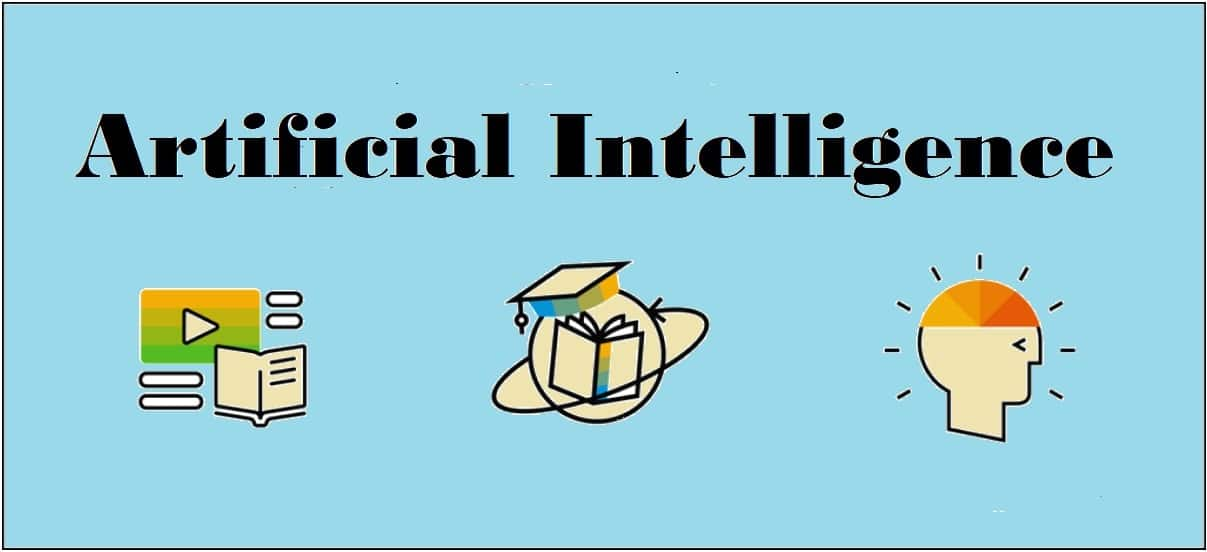## Unsupervised Learning Clustering

Unsupervised machine learning algorithms do not have any supervisor to provide any sort of guidance. That is why they are closely aligned with what some call true artificial intelligence. In unsupervised learning, there would be no correct answer and no teacher for the guidance. Algorithms need to discover the interesting pattern in data for learning.

### Clustering:

Basically, it is a type of unsupervised learning method and a common technique for statistical data analysis used in many fields. Clustering mainly is a task of dividing the set of observations into subsets, called clusters, in such a way that observations in the same cluster are similar in one sense and they are dissimilar to the observations in other clusters. In simple words, we can say that the main goal of clustering is to group the data on the basis of similarity and dissimilarity.

For example, the following diagram shows similar kind of data in different clusters −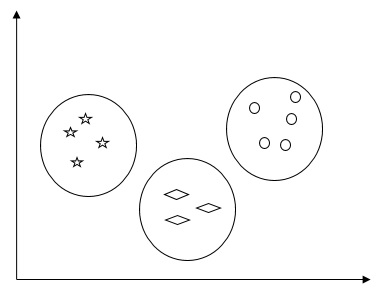### Algorithms for Clustering the Data

Following are a few common algorithms for clustering the data −

### K-Means algorithm

K-means clustering algorithm is one of the well-known algorithms for clustering the data. We need to assume that the numbers of clusters are already known. This is also called flat clustering. It is an iterative clustering algorithm. The steps given below need to be followed for this algorithm:

Step 1 − We need to specify the desired number of K subgroups.

Step 2 − Fix the number of clusters and randomly assign each data point to a cluster. Or in other words we need to classify our data based on the number of clusters.

In this step, cluster centroids should be computed.

As this is an iterative algorithm, we need to update the locations of K centroids with every iteration until we find the global optima or in other words the centroids reach at their optimal locations.

The following code will help in implementing K-means clustering algorithm in Python. We are going to use the Scikit-learn module.

[wpsbx_html_block id=1891]

Let us import the necessary packages −

import matplotlib.pyplot as plt
import seaborn as sns; sns.set()
import numpy as np
from sklearn.cluster import KMeans

The following line of code will help in generating the two-dimensional dataset, containing four blobs, by using make_blob from the sklearn.dataset package.

from sklearn.datasets.samples_generator import make_blobs

X, y_true = make_blobs(n_samples = 500, centers = 4,
cluster_std = 0.40, random_state = 0)

We can visualize the dataset by using the following code −

plt.scatter(X[:, 0], X[:, 1], s = 50);
plt.show()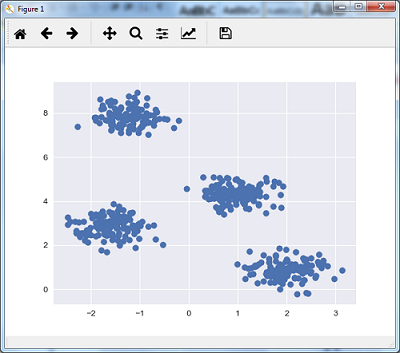Here, we are initializing kmeans to be the KMeans algorithm, with the required parameter of how many clusters (n_clusters).

kmeans = KMeans(n_clusters = 4)

We need to train the K-means model with the input data.

kmeans.fit(X)
y_kmeans = kmeans.predict(X)
plt.scatter(X[:, 0], X[:, 1], c = y_kmeans, s = 50, cmap = 'viridis')

centers = kmeans.cluster_centers_

The code given below will help us plot and visualize the machine’s findings based on our data, and the fitment according to the number of clusters that are to be found.

plt.scatter(centers[:, 0], centers[:, 1], c = 'black', s = 200, alpha = 0.5);
plt.show()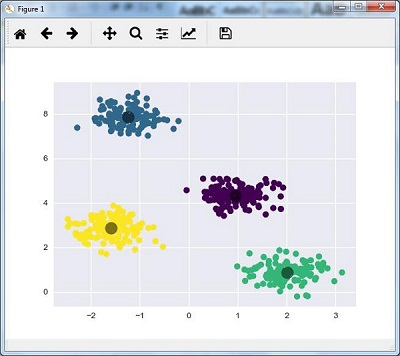### Mean Shift Algorithm

It is another popular and powerful clustering algorithm used in unsupervised learning. It does not make any assumptions hence it is a non-parametric algorithm. It is also called hierarchical clustering or mean shift cluster analysis. Followings would be the basic steps of this algorithm −

• First of all, we need to start with the data points assigned to a cluster of their own.
• Now, it computes the centroids and update the location of new centroids.
• By repeating this process, we move closer the peak of cluster i.e. towards the region of higher density.
• This algorithm stops at the stage where centroids do not move anymore.

With the help of following code we are implementing Mean Shift clustering algorithm in Python. We are going to use Scikit-learn module.

Let us import the necessary packages −

import numpy as np
from sklearn.cluster import MeanShift
import matplotlib.pyplot as plt
from matplotlib import style
style.use("ggplot")

The following code will help in generating the two-dimensional dataset, containing four blobs, by using make_blob from the sklearn.dataset package.

from sklearn.datasets.samples_generator import make_blobs

We can visualize the dataset with the following code

centers = [[2,2],[4,5],[3,10]]
X, _ = make_blobs(n_samples = 500, centers = centers, cluster_std = 1)
plt.scatter(X[:,0],X[:,1])
plt.show()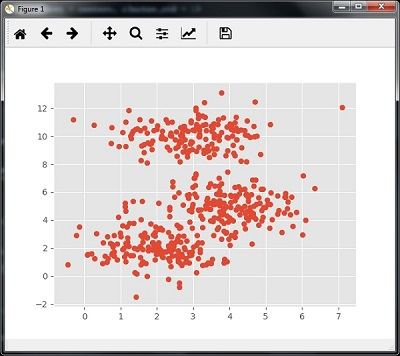Now, we need to train the Mean Shift cluster model with the input data.

ms = MeanShift()
ms.fit(X)
labels = ms.labels_
cluster_centers = ms.cluster_centers_

The following code will print the cluster centers and the expected number of cluster as per the input data −

print(cluster_centers)
n_clusters_ = len(np.unique(labels))
print("Estimated clusters:", n_clusters_)
[[ 3.23005036 3.84771893]
[ 3.02057451 9.88928991]]
Estimated clusters: 2

The code given below will help plot and visualize the machine’s findings based on our data, and the fitment according to the number of clusters that are to be found.

colors = 10*['r.','g.','b.','c.','k.','y.','m.']
for i in range(len(X)):
plt.plot(X[i], X[i], colors[labels[i]], markersize = 10)
plt.scatter(cluster_centers[:,0],cluster_centers[:,1],
marker = "x",color = 'k', s = 150, linewidths = 5, zorder = 10)
plt.show()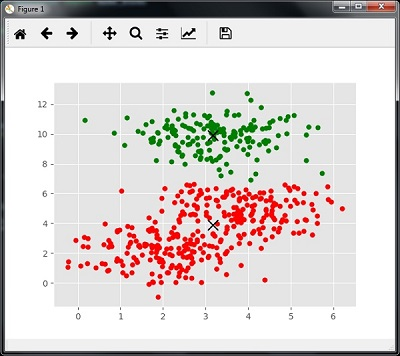### Measuring the Clustering Performance

The real world data is not naturally organized into number of distinctive clusters. Due to this reason, it is not easy to visualize and draw inferences. That is why we need to measure the clustering performance as well as its quality. It can be done with the help of silhouette analysis.

### Silhouette Analysis

This method can be used to check the quality of clustering by measuring the distance between the clusters. Basically, it provides a way to assess the parameters like number of clusters by giving a silhouette score. This score is a metric that measures how close each point in one cluster is to the points in the neighboring clusters.

### Analysis of silhouette score

The score has a range of [-1, 1]. Following is the analysis of this score −

• Score of +1 − Score near +1 indicates that the sample is far away from the neighboring cluster.
• Score of 0 − Score 0 indicates that the sample is on or very close to the decision boundary between two neighboring clusters.
• Score of -1 − Negative score indicates that the samples have been assigned to the wrong clusters.

### Calculating Silhouette Score

In this section, we will learn how to calculate the silhouette score.

Silhouette score can be calculated by using the following formula −

$$silhouette score = \frac{\left ( p-q \right )}{max\left ( p,q \right )}$$

Here, 𝑝 is the mean distance to the points in the nearest cluster that the data point is not a part of. And, 𝑞 is the mean intra-cluster distance to all the points in its own cluster.

For finding the optimal number of clusters, we need to run the clustering algorithm again by importing the metrics module from the sklearn package. In the following example, we will run the K-means clustering algorithm to find the optimal number of clusters −

Import the necessary packages as shown −

import matplotlib.pyplot as plt
import seaborn as sns; sns.set()
import numpy as np
from sklearn.cluster import KMeans

With the help of the following code, we will generate the two-dimensional dataset, containing four blobs, by using make_blob from the sklearn.dataset package.

from sklearn.datasets.samples_generator import make_blobs

X, y_true = make_blobs(n_samples = 500, centers = 4, cluster_std = 0.40, random_state = 0)

Initialize the variables as shown −

scores = []
values = np.arange(2, 10)

We need to iterate the K-means model through all the values and also need to train it with the input data.

for num_clusters in values:
kmeans = KMeans(init = 'k-means++', n_clusters = num_clusters, n_init = 10)
kmeans.fit(X)

Now, estimate the silhouette score for the current clustering model using the Euclidean distance metric −

score = metrics.silhouette_score(X, kmeans.labels_,
metric = 'euclidean', sample_size = len(X))

The following line of code will help in displaying the number of clusters as well as Silhouette score.

print("\nNumber of clusters =", num_clusters)
print("Silhouette score =", score)
scores.append(score)

You will receive the following output −

Number of clusters = 9
Silhouette score = 0.340391138371

num_clusters = np.argmax(scores) + values
print('\nOptimal number of clusters =', num_clusters)


Now, the output for optimal number of clusters would be as follows −

Optimal number of clusters = 2


### Finding Nearest Neighbors

The concept of finding nearest neighbors may be defined as the process of finding the closest point to the input point from the given dataset. The main use of this KNN)K-nearest neighbors) algorithm is to build classification systems that classify a data point on the proximity of the input data point to various classes.

The Python code given below helps in finding the K-nearest neighbors of a given data set −

Import the necessary packages as shown below. Here, we are using the NearestNeighbors module from the sklearn package

import numpy as np
import matplotlib.pyplot as plt
from sklearn.neighbors import NearestNeighbors

Let us now define the input data −

A = np.array([[3.1, 2.3], [2.3, 4.2], [3.9, 3.5], [3.7, 6.4], [4.8, 1.9],
[8.3, 3.1], [5.2, 7.5], [4.8, 4.7], [3.5, 5.1], [4.4, 2.9],])

Now, we need to define the nearest neighbors −

k = 3

We also need to give the test data from which the nearest neighbors is to be found −

test_data = [3.3, 2.9]

The following code can visualize and plot the input data defined by us −

plt.figure()
plt.title('Input data')
plt.scatter(A[:,0], A[:,1], marker = 'o', s = 100, color = 'black')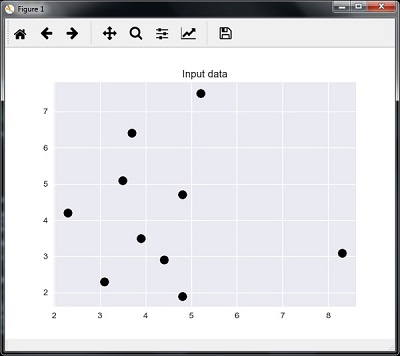Now, we need to build the K Nearest Neighbor. The object also needs to be trained

knn_model = NearestNeighbors(n_neighbors = k, algorithm = 'auto').fit(X)
distances, indices = knn_model.kneighbors([test_data])

Now, we can print the K nearest neighbors as follows

print("\nK Nearest Neighbors:")
for rank, index in enumerate(indices[:k], start = 1):
print(str(rank) + " is", A[index])

We can visualize the nearest neighbors along with the test data point

plt.figure()
plt.title('Nearest neighbors')
plt.scatter(A[:, 0], X[:, 1], marker = 'o', s = 100, color = 'k')
plt.scatter(A[indices][:][:, 0], A[indices][:][:, 1],
marker = 'o', s = 250, color = 'k', facecolors = 'none')
plt.scatter(test_data, test_data,
marker = 'x', s = 100, color = 'k')
plt.show()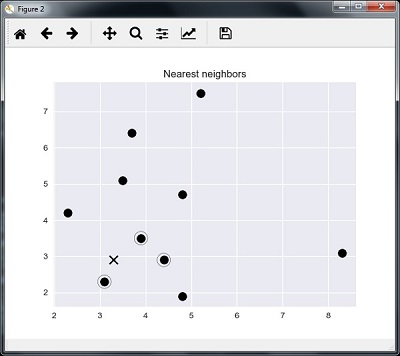### Output

K Nearest Neighbors

1 is [ 3.1 2.3]
2 is [ 3.9 3.5]
3 is [ 4.4 2.9]


### K-Nearest Neighbors Classifier

A K-Nearest Neighbors (KNN) classifier is a classification model that uses the nearest neighbors algorithm to classify a given data point. We have implemented the KNN algorithm in the last section, now we are going to build a KNN classifier using that algorithm.

### Concept of KNN Classifier

The basic concept of K-nearest neighbor classification is to find a predefined number, i.e., the ‘k’ − of training samples closest in distance to a new sample, which has to be classified. New samples will get their label from the neighbors itself. The KNN classifiers have a fixed user defined constant for the number of neighbors which have to be determined. For the distance, standard Euclidean distance is the most common choice. The KNN Classifier works directly on the learned samples rather than creating the rules for learning. The KNN algorithm is among the simplest of all machine learning algorithms. It has been quite successful in a large number of classification and regression problems, for example, character recognition or image analysis.

Example

We are building a KNN classifier to recognize digits. For this, we will use the MNIST dataset. We will write this code in the Jupyter Notebook.

Import the necessary packages as shown below.

Here we are using the KNeighborsClassifier module from the sklearn.neighbors package −

from sklearn.datasets import *
import pandas as pd
%matplotlib inline
from sklearn.neighbors import KNeighborsClassifier
import matplotlib.pyplot as plt
import numpy as np

The following code will display the image of digit to verify what image we have to test −

def Image_display(i):
plt.imshow(digit['images'][i],cmap = 'Greys_r')
plt.show()

Now, we need to load the MNIST dataset. Actually there are total 1797 images but we are using the first 1600 images as training sample and the remaining 197 would be kept for testing purpose.

digit = load_digits()
digit_d = pd.DataFrame(digit['data'][0:1600])

Now, on displaying the images we can see the output as follows −

Image_display(0)


### Image_display(0)

Image of 0 is displayed as follows −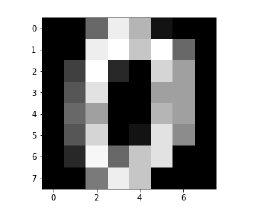### Image_display(9)

Image of 9 is displayed as follows −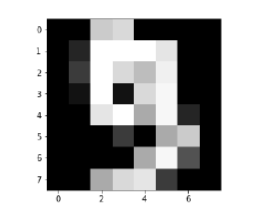### digit.keys()

Now, we need to create the training and testing data set and supply testing data set to the KNN classifiers.

train_x = digit['data'][:1600]
train_y = digit['target'][:1600]
KNN = KNeighborsClassifier(20)
KNN.fit(train_x,train_y)

The following output will create the K nearest neighbor classifier constructor −

KNeighborsClassifier(algorithm = 'auto', leaf_size = 30, metric = 'minkowski',
metric_params = None, n_jobs = 1, n_neighbors = 20, p = 2,
weights = 'uniform')


We need to create the testing sample by providing any arbitrary number greater than 1600, which were the training samples.

test = np.array(digit['data'])
test1 = test.reshape(1,-1)
Image_display(1725)

### Image_display(6)

Image of 6 is displayed as follows −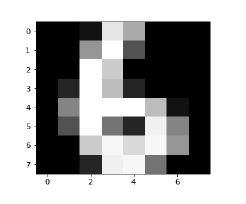Now we will predict the test data as follows −

KNN.predict(test1)

The above code will generate the following output −

array()


Now, consider the following −

digit['target_names']

The above code will generate the following output −

array([0, 1, 2, 3, 4, 5, 6, 7, 8, 9])